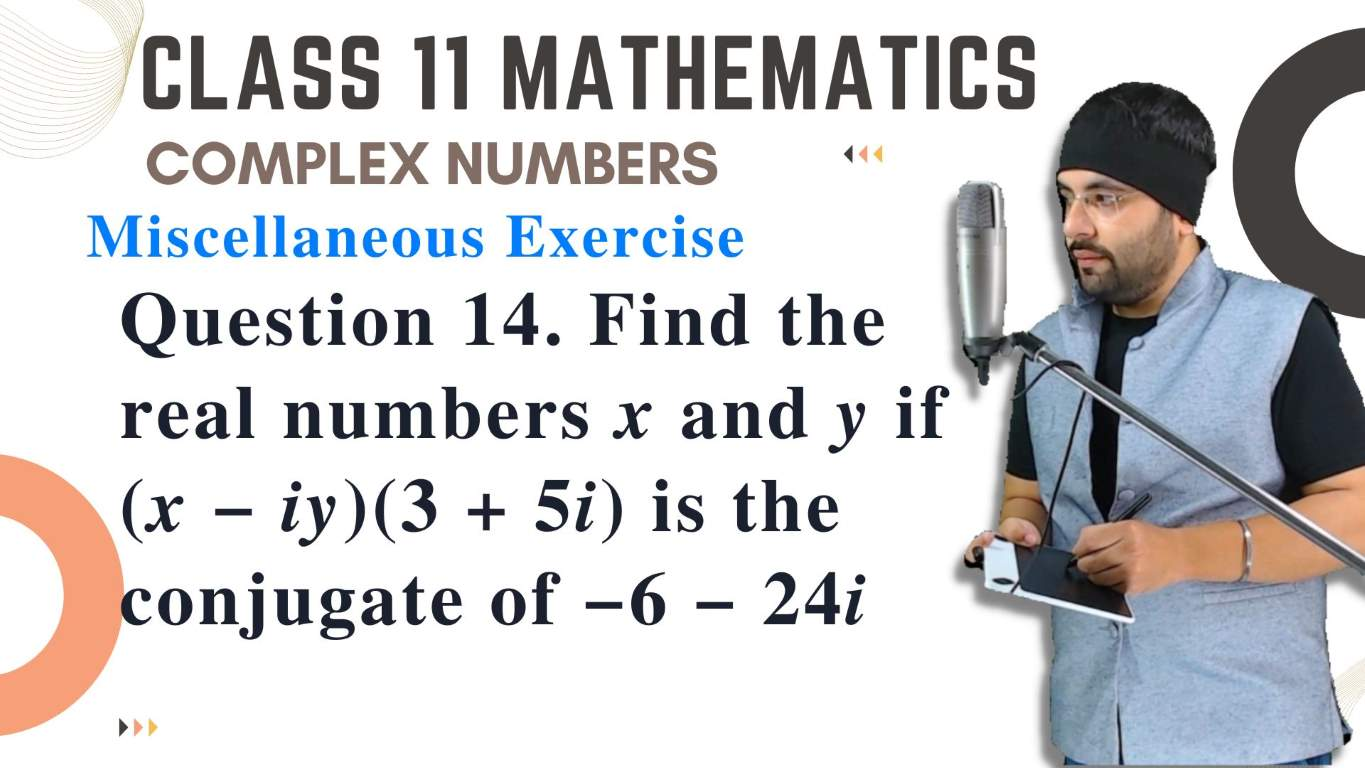03:02

## Question 14 Find the real numbers x and y if (x−iy)(3+5i) is the conjugate of −6−24i

NCERT Miscellaneous Exercise Question 14. Find the real numbers x and y if {\displaystyle (x-iy)(3+5i) } is the conjugate of {\displaystyle -6-24i } .05:43

## Example 12 Find the conjugate

NCERT Chapter 5 Complex Numbers Example 12 Find the conjugate of {\displaystyle \frac{(3-2i)(2+3i)}{(1+2i)(2-i)} }04:43

## Find the modulus and the arguments of each of the complex numbers in

NCERT Exercise 5.2 Find the modulus and the arguments of each of the complex numbers in Exercises 1 to 2. Question - 1: {\displaystyle z=-1-i \sqrt{3} } .

M A T H Y U G